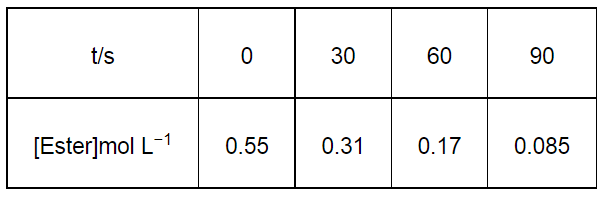# In a pseudo first order hydrolysis of ester in water, the following results were obtained:`
Question:

In a pseudo first order hydrolysis of ester in water, the following results were obtained:(i) Calculate the average rate of reaction between the time interval 30 to 60 seconds.

(ii) Calculate the pseudo first order rate constant for the hydrolysis of ester.

Solution:

(i) Average rate of reaction between the time interval, 30 to 60 seconds, $=\frac{d[\text { Ester }]}{d t}$

$=\frac{0.31-0.17}{60-30}$

$=\frac{0.14}{30}$

= 4.67 × 10−3 mol L−1 s−1

(ii) For a pseudo first order reaction,

$k=\frac{2.303}{t} \log \frac{[\mathrm{R}]_{0}}{[\mathrm{R}]}$

For $t=30 \mathrm{~s}, k_{1}=\frac{2.303}{30} \log \frac{0.55}{0.31}$

= 1.911 × 10−2 s−1

For $t=60 \mathrm{~s}, k_{2}=\frac{2.303}{60} \log \frac{0.55}{0.17}$

= 1.957 × 10−2 s−1

For $t=90 \mathrm{~s}, k_{3}=\frac{2.303}{90} \log \frac{0.55}{0.085}$

= 2.075 × 10−2 s−1

Then, average rate constant, $k=\frac{k_{1}+k_{2}+k_{3}}{3}$

$=\frac{\left(1.911 \times 10^{-2}\right)+\left(1.957 \times 10^{-2}\right)+\left(2.075 \times 10^{-2}\right)}{3}$

$=1.98 \times 10^{-2} \mathrm{~s}^{-1}$